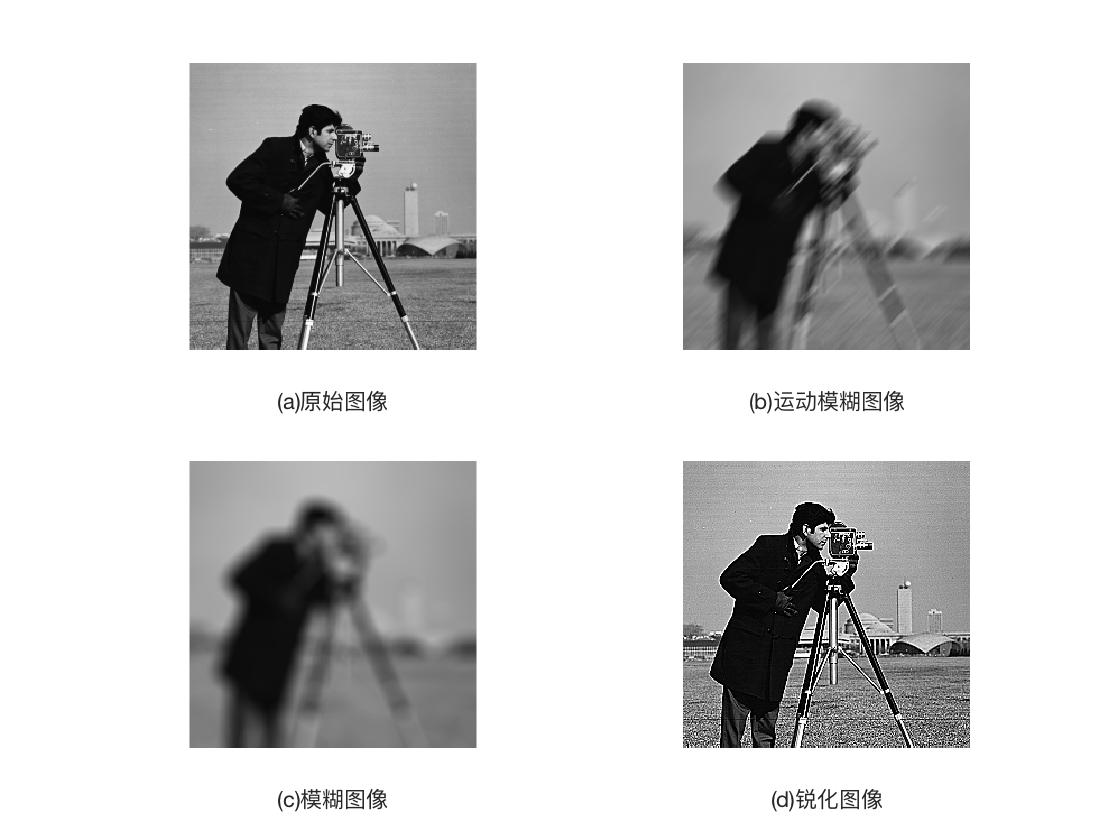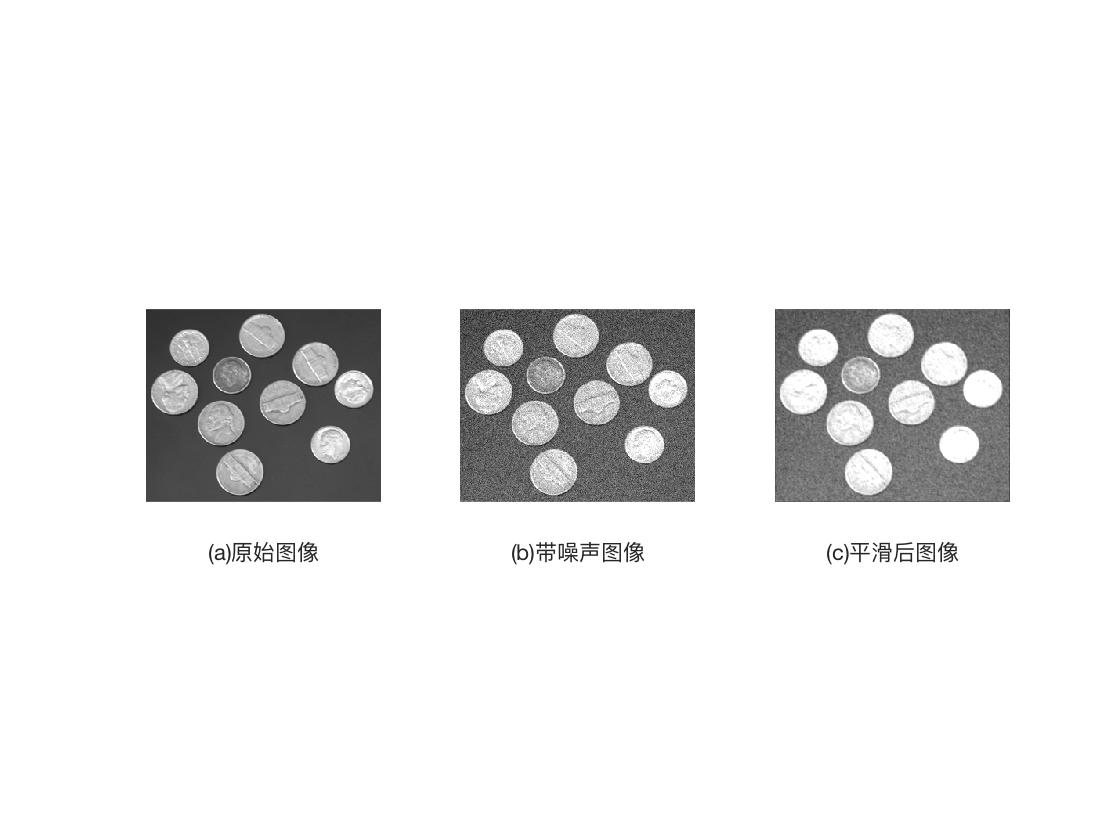• matlab开发-图像平均nguingboxfilter。不带内置函数的盒形滤波器图像平均
• 本资源是一个通过 matlab求一副图像平均值的代码，可直接运行
• <p>matlab图像的算术平均像素值怎么求啊，就是需要求一个图像里面各个点的算术平均像素值，得到的应该是一个矩阵，求大佬们指点指点！！！</p>
• 求大神指导如何用matlab求解一副图像平均梯度 比如这幅图： [img=https://img-bbs.csdn.net/upload/201305/07/1367924081_720755.jpg][/img]
• 读入视频中同一场景的数帧图像，然后将图像叠加，通过求平均值来降低图像中的噪声。 matlab程序实现 输入：.avi视频 输出：叠加求平均后的图像 Obj = VideoReader('zgn1.avi'); save_path = '.\'; index...
读入视频中同一场景的数帧图像，然后将图像叠加，通过求平均值来降低图像中的噪声。
matlab程序实现
输入：.avi视频
输出：叠加求平均后的图像

Obj = VideoReader('zgn1.avi');
save_path = '.\';
index_start = 1;
index_end = 50;

nFrames = Obj.NumberOfFrames;
vidHeight = Obj.Height;
vidWidth = Obj.Width;

frame_start = index_start;
frame_end = index_end;

if frame_num < index_start
disp('total frame < parameter index_start');
return;
end

if frame_num < index_end
frame_end = frame_num;
end

% 预分配空间 Preallocate movie structure.
mov =  struct('cdata',zeros(vidHeight,vidWidth,3,'uint8'),...
'colormap',[]);

% Read one frame at a time.
for k = frame_start : frame_end
mov.cdata = read(Obj,k);
file_data = frame2im( mov );

if ( 3 == ndims( file_data ) )
file_data = rgb2gray( file_data );
end

roi_data = file_data;

if k == 1
I = double(roi_data);
else
I = I + double(roi_data);
end
end

I2 = uint8(I/(index_end-index_start+1));
imwrite(I2, 'out.png');
figure;
imshow(I2);


展开全文• matlab开发-带权重的图像平均化任务。加权掩模滤波器图像平均
• clc; clear; close all; img = imread('crop/1.png'); img = im2double(img); [~,~,imxy] = my_gradient(img); s1 = mean(imxy(:)); disp(s1); function [imx,imy,imnorm] = my_...imy = [im(end,:,:) - im(1.
clc;
clear;
close all;

img = imread('crop/1.png');
img = im2double(img);

[~,~,imxy] = my_gradient(img);
s1 = mean(imxy(:));
disp(s1);

function [imx,imy,imnorm] = my_gradient(im)

imx = [im(:,end,:) - im(:, 1,:),-diff(im,1,2)];
imy = [im(end,:,:) - im(1, :,:);-diff(im,1,1)];
imnorm = sqrt(imx.^2 + imy.^2);

end


展开全文• matlab开发-图像增强平均图像质量以提高对比度版权保护。基于图像增强平均直方图均衡化的对比度增强亮度保持
• matlab开发-平均阈值局部图像阈值。meanthresh执行简单的本地图像阈值。
• 基于加权平均融合规则的图像融合MATLAB算法 含融合源图像
• Matlab图像增强程序分享-图像增强.m ⑴直方图均衡化（histogram equalization）， ⑵直方图匹配（histogram matching）， ⑶邻域平均（neighborhood averaging）， ⑷局域增强（local enhancement）， ⑸...
• 邻域平均的线性平滑滤波法实现降噪 winner滤波法实现降噪 线性锐化滤波 非线性锐滤波 Buterworth低通滤波器 Buterworth高通滤波器 灰度分层法彩色图像的实现 空间域灰度级－彩色变换的方法，进行图像...
• 一、调整灰度范围：y=k*x...1、原图像矩阵灰度值范围以及平均值获取 maxIm=double（max（max（inputIm））），inputIm是原图像矩阵 minIm=double（min（min（inputIm））） [row col]=size(inputIm) 获取矩阵行列...
一、调整灰度范围：y=k*x+b；y是调整后的图像矩阵，x是原图像矩阵。设调整后的灰度范围是【rangehigh-rangelow】。

1、原图像矩阵灰度值范围以及平均值获取

maxIm=double（max（max（inputIm））），inputIm是原图像矩阵

minIm=double（min（min（inputIm）））

[row col]=size(inputIm) 获取矩阵行列数

for i=1：row

for j=1：col

sum=sum+inputIm（i，j）；

end

end      %获取矩阵之和

mean=sum/(row*col); %获取平均值，即图像像素点只要集中在该值附近

k=(rangehigh-rangelow)/(maxIm-minIm);

b=rangehigh-maxIm*k;

另外需要说明的是，image函数可以显示色彩图，而imshow函数貌似只能显示灰度图，详细可以查询matlab help


展开全文• ## matlab图像处理——平滑滤波

万次阅读 多人点赞 2018-11-01 20:52:00
平滑滤波——matlab图像处理 平滑滤波的目的是消除或尽量减少噪声，改善图像的质量。假设加性噪声是随机独立分布，这样利用图像像素领域的平均或加权平均即可有效地抑制噪声干扰。从信号分析的观点来看，图像平滑...
平滑滤波——matlab图像处理
平滑滤波的目的是消除或尽量减少噪声，改善图像的质量。假设加性噪声是随机独立分布，这样利用图像像素领域的平均或加权平均即可有效地抑制噪声干扰。从信号分析的观点来看，图像平滑本质上是低能滤波，信号的低频部分可通过，高频的噪声信号被阻截。但由于图像边缘也处于高频部分，这样往往带来另一个问题：在对图像进行平滑处理时，往往对图像的细化造成一定程度的损坏。
领域运算可用领域与模版的卷积得到，这极大地方便了计算。
MATLAB中提供的imfliter函数用于实现图像的平滑处理，其调用格式如下。
B=imfliter（A，H）：使用多维滤波器H对图像A进行滤波（平滑）。参数A可以是任意维的二值或非奇异数值型矩阵。参数H为矩阵，表示滤波器。H常由函数fspecial输出得到。返回值B与A的维数相同。
B=imfliter（A,H，optional1，optional2，…）

参数类型
说明

X
输入图像的外边界通过X来扩展，X默认值为0

symmetric
输入图像的外部边界通过镜像反射其内部边界来扩展

circular
输入图像的别界通过假设输入图像为周期函数来扩展

relicate
输入图像的外部别界通过复制内部别界的值来扩展

same
输入和输出图像大小相等，默认操作

full
输出图像比输入图像大

corr
使用相关进行滤波（平滑）

conv
使用卷积进行滤波（平滑）

matlab中提供的fspecial函数用于创建二维滤波器：
h=fspecial（type）：
type可以是：average，disk，gaussian，laplacian，log，motion，prewitt，sobel，unsharp。
h=fspecial（type，parameters）：创建指定类型和指定参数的二维滤波器h。参数parameters为与滤波器有关的参数。
parameters可以是：n，radius，（hsize，sigma），alpha，（n，sigma），（len，theta）。
clear all;
I = imread('cameraman.tif');
subplot(2,2,1);imshow(I);
xlabel('(a)原始图像');
H = fspecial('motion',20,45);
MotionBlur = imfilter(I,H,'replicate');
subplot(2,2,2);imshow(MotionBlur);
xlabel('(b)运动模糊图像');
H=fspecial('disk',10);
blurred = imfilter(I,H,'replicate');
subplot(2,2,3);imshow(blurred);
xlabel('(c)模糊图像');
H=fspecial('unsharp');
sharpened = imfilter(I,H,'replicate');
subplot(2,2,4);imshow(sharpened);
xlabel('(d)锐化图像');对含有高斯噪声的图像进行平滑处理。
clear all;
I = imread('coins.png');
Inoised = imnoise(I,'gaussian',0.1,0.005);%对图像进行高斯噪声加噪
%制定卷积核
h=ones(3,3)/5;
h(1,1) = 0;
h(1,3) = 0;
h(3,1) = 0;
h(1,3) = 0;
%平滑运算
I2=imfilter(Inoised,h);
subplot(1,3,1);imshow(I);
xlabel('(a)原始图像');
subplot(1,3,2);imshow(Inoised);
xlabel('(b)带噪声图像');
subplot(1,3,3);imshow(I2);
xlabel('(c)平滑后图像');有问题还请多多指教。刚刚入门。谢谢各位大牛。


展开全文平滑滤波
• If the only way is to average them after load all the frames into matlab, how should I average over 1000 frames? Thanks. 解决方案 try the following: a=zeros(512); for i=1:1000 a=a+frame(i); end a=a/...
• 图像的最大灰度、最小灰度、以及平均灰度。 代码如下： % BY SCOTT % Count max gray and min gray 。。。。。。。
• Matlab图像增强程序分享-数字图像处理实验二.doc ⑴直方图均衡化（histogram equalization）， ⑵直方图匹配（histogram matching）， ⑶邻域平均（neighborhood averaging）， ⑷局域增强（local enhancement...
• 二、研究内容本文主要研究分析邻域平均法、中值滤波法及小波变换法的图像去噪算法。首先介绍图像处理应用时的常用函数及其用法；其次详细阐述了三种去噪算法原理及特点；最后运用Matlab软件对一张含噪图片（含高斯...
• ## matlab图像的增强

千次阅读 2018-09-26 15:16:56
简单平滑是图像增强处理中最基本的方法之一，主要是利用了卷积运算对领域的像素灰度进行平均，从而达到减少图像中噪声的影响，降低图像对比度。PS：图像的质量会严重下降。 |1| 1 |1 |1| 1 |1 |1| 1 |1 这种矩阵...gaussian
• 实验目的 ...3、 运用for循环，将100幅加有噪声的图像进行相加并求其平均值,显示求平均图像。 4、 将图像分别放大1.5倍和缩小0.8倍，插值方法使用双线性插值法。将图像顺时针旋转45度，显示旋转后
• matlab实现平均梯度，对图像融合结果进行评价
• 均值滤波是典型的线性滤波算法，它是指在图像上对目标像素给一个模板，该模板包括了其周围的临近像素（以目标像素为中心的周围8个像素，构成一个滤波模板，即去掉目标像素本身），再用模板中的全体像素的平均值来...
• ## Matlab图像处理—锐化滤波器

万次阅读 多人点赞 2019-03-23 12:33:36
锐化滤波器 锐化处理的主要目的是，突出灰度的过渡部分，增强图像中的细节。空间域像素邻域平均法可以使图像变模糊，均值处理与积分类似锐化滤波器
• ## Matlab图像代数运算

千次阅读 2014-07-26 11:07:14
图像的代数运算不但可以作为复杂图像处理的预处理步骤，还有其它许多用途，如：加法对同一场景的多幅图像平均，可以有效地降低具有叠加性质的随机噪声等。 1.点运算 所谓的点运算是指，对一幅输入图像而...
• 有关于求取图像平均梯度的matlab M 文件；可以为你省去那么一点时间。 ———共享改变未来！——— 有关于求取图像平均梯度的matlab M 文件；可以为你省去那么一点时间。 ———共享改变未来！———
• 也可以把同一景物的多重影像加起来求平均，以便减少图像的随机噪声。 Z=imadd(X,Y),对X 和Y数组中对应元素相加，返回值Z和X,Y大小一致。如果Y为标量，则X数组中每个元素加上这个变量。该函数类似矩阵的加法运算，但...
• 均值滤波是典型的线性滤波算法，它是指在图像上对目标像素给一个模板，该模板包括了其周围的临近像素（以目标象素为中心的周围8个像素，构成一个滤波模板，即去掉目标像素本身），再用模板中的全体像素的平均值来...
• 一、评价指标分类 对于融合图像，其评价指标...2.1 平均梯度算法 function outval=ftidu(img) A=double(img); [r,c]=size(A); [dzdx,dzdy]=gradient(A); %分别求X的梯度和Y的梯度 s=sqrt((dzdx.^2+dzdy.^2)./2...

# matlab图像平均matlab 订阅